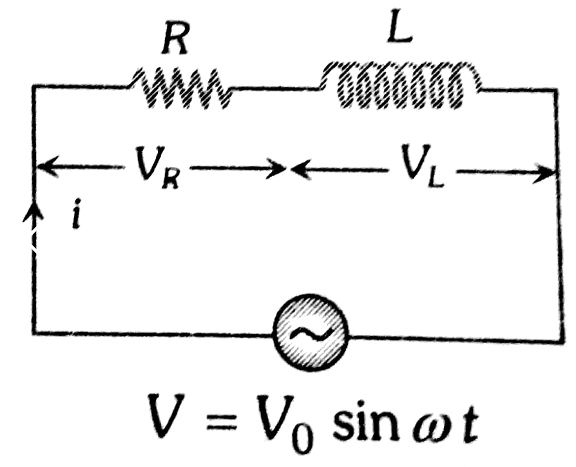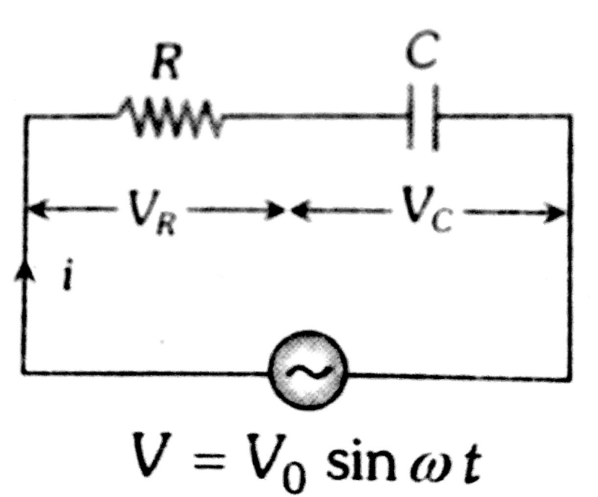# In a series LCR circuit R = 200 and the voltage and the frequency of the main supply is 220 V and 50 Hz respectively. On taking out the capacitance from the circuit the current lags behind the voltage by 300. On taking out the inductor from the circuit the current leads the voltage by 300. The power dissipated in the LCR circuit is Option 1) 242 W Option 2) 305 W Option 3) 210 W Option 4) zero W

D Divya Saini

As we learnt in

R.L Circuit Voltage -

- whereinRC Circuit Voltage -

- whereinR=500

(When capacitance is taken out)

---------------(1)

on taking out inductor, current lead by

-----------------------(2)

z=R

power dissipated in the circuit

Option 1)

242 W

This option is correct

Option 2)

305 W

This option is incorrect

Option 3)

210 W

This option is incorrect

Option 4)

zero W

This option is incorrect

Exams
Articles
Questions